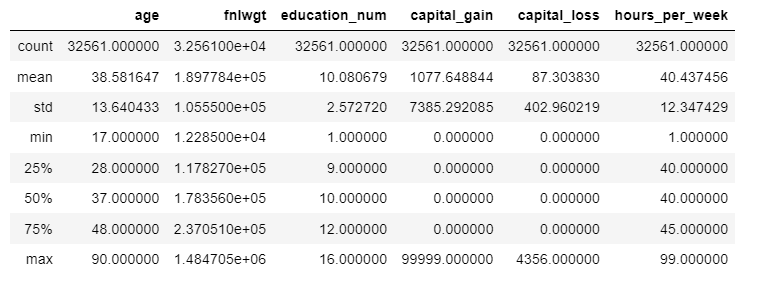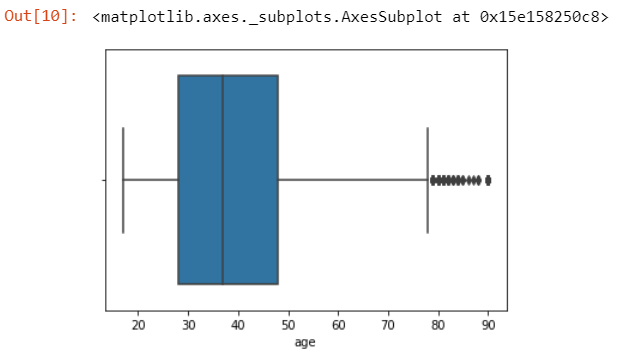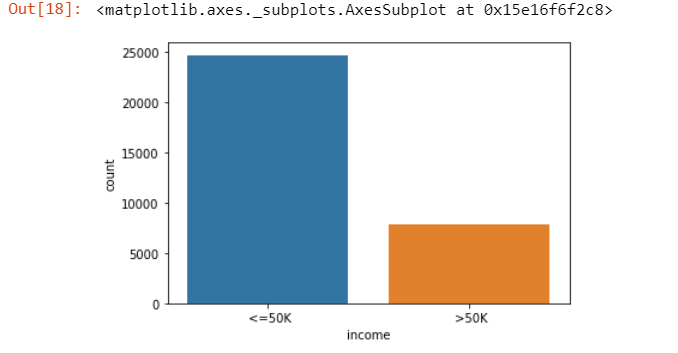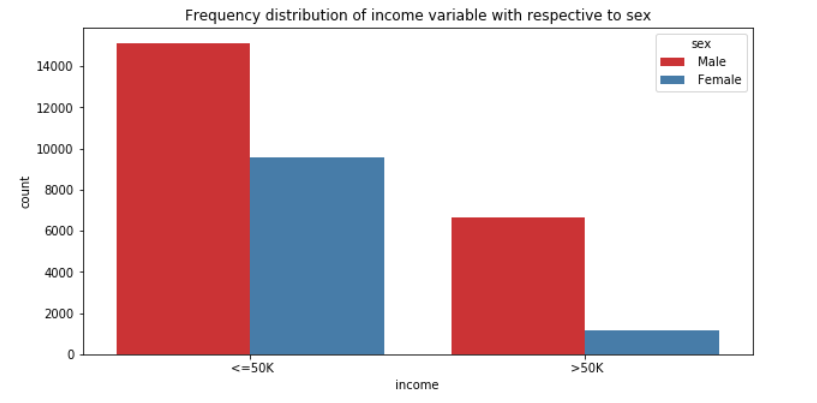Search

# Exploratory Data Analysis (EDA) Assignment Help| EDA of Income Evaluation Data

The concept of this project is to analyze the income evaluation of the people who belong to different countries. Compare the income of the people who belong to different sectors, country, age, occupation etc. The performance of can be evaluated by gender wise and age wise. It primarily aims at learning the various factors that can help my evaluation process.

The information provided in the income evaluation dataset is used to give better services, improve the quality of life and solve the existing problem of people. The analysis is the process to identify the problems and solutions of that problem.

Introduction

The census is a special, wide-range activity that occurs once a decade in the entire country. The purpose is to gather information about the general population, in order to present a full and reliable picture of the population in the country. The information collected includes data on age, gender, country of origin, marital status, occupation, how many hours they work in a week, education, employment, etc.

This information makes it possible to plan better services, improve the quality of life and solve existing problems. Statistical information, which serves as the basis for constructing planning forecasts, is essential for the democratic process since it enables the citizens to examine the decisions made by the government and local authorities, and decide whether they serve the public they are meant to help.

Dataset

### Implementation

Import Libraries

```#import Libraries
import pandas as pd
import seaborn as sns
import matplotlib.pyplot as plt
import numpy as np

```

```#read data

OutputView the dimension of the data set

```# print the shape
print('The shape of the dataset : ', df.shape)```

output:

The shape of the dataset : (32561, 15)

Describe the data

View the statistical properties of the dataset

```# describe the data
df.describe()```

Output:### View the summary of data

info() function get a concise summary of the data. It comes really handy when doing exploratory analysis of the data. To get a quick overview of the dataset we use the info() function.

`df.info()`

Output:

<class 'pandas.core.frame.DataFrame'> RangeIndex: 32561 entries, 0 to 32560 Data columns (total 15 columns): age 32561 non-null int64 workclass 32561 non-null object fnlwgt 32561 non-null int64 education 32561 non-null object education_num 32561 non-null int64 marital_status 32561 non-null object occupation 32561 non-null object relationship 32561 non-null object race 32561 non-null object sex 32561 non-null object capital_gain 32561 non-null int64 capital_loss 32561 non-null int64 hours_per_week 32561 non-null int64 native_country 32561 non-null object income 32561 non-null object dtypes: int64(6), object(9) memory usage: 3.7+ MB

Check the null value are in dataset

```check_null_value = df.isnull()
sns.heatmap(check_null_value,yticklabels=False,cbar=False,cmap='viridis')```Plotting graph of correlation

```fig = plt.figure(figsize=(10,5))
sns.heatmap(df.corr())```

OutputBox Plot

Some points are plot between 80 and 90 they are outliers.

```import matplotlib.pyplot as plt
sns.boxplot(x=df['age'])```

OutputScatter plot

In the graph clearly show maximum number of people capital Gain is less than 2000

```fig, ax = plt.subplots(figsize=(16,8))
ax.scatter(df['age'], df['capital_gain'])
ax.set_xlabel('Proportion of Age')
ax.set_ylabel('Proportion of Capital Gain')
plt.show()```

Output:Bar Plot(Using Single Input Variable)

Plotting the graph the number of people by their income

`sns.countplot(x='income',data=df)`

Output:Pie Chart

There are 75.9 % people income are less the 50000 and 24.1% people income is greater than 50000

```labels = ["<=50k",'>=50k']
values = df['income'].value_counts().values

fig1, ax1 = plt.subplots()
colors = ['red', 'lightskyblue']
plt.show()```

Output:Bar plot(Using Two input variable)

In the bar blot we can see there are above 14000 male income and around 10000 female income is less than 50K. Around 6000 male income and around 1000 female income is greater than 50k

```f, ax = plt.subplots(figsize=(10, 5))
ax = sns.countplot(x="income", hue="sex", data=df, palette="Set1")
ax.set_title("Frequency distribution of income variable with respective to sex")
plt.show()```

Output:Label Encoder

### Converting the categorical data into numberical data

```from sklearn.model_selection import train_test_split
from sklearn.preprocessing import LabelEncoder

#build label encoder
lbl_enc = LabelEncoder()
df["income_label"] = lbl_enc.fit_transform(df[["income"]])

Output:Declaring the feature vector and target variable

```X = df.iloc[:,]
y = df['income_label']```

Split data into separate training and test sets

```# Create training and test sets
X_train, X_test, y_train, y_test = train_test_split(X, y,test_size = 0.1)```

Build the Random forest classfier model

Calculating: Train and test data Accuracy : showing the accuracy of model Confusion Matrix : Describe the performance of classification of model Classification Report

```#Logistic Regression

from sklearn.linear_model import LogisticRegression
from sklearn.metrics import accuracy_score
from sklearn.metrics import confusion_matrix,classification_report

## Build lostictic regression model
model = LogisticRegression()
model.fit(X_train,y_train)
y_train_pred = model.predict(X_train)
y_test_pred = model.predict(X_test)

#Accuracy confusion matrix  and classification report
print("Train Set Accuracy:"+str(accuracy_score(y_train_pred,y_train)*100))
print("Test Set Accuracy:"+str(accuracy_score(y_test_pred,y_test)*100))
print("\nConfusion Matrix:\n%s"%confusion_matrix(y_test_pred,y_test))
print("\nClassification Report:\n%s"%classification_report(y_test_pred,y_test))```

Output:

```Train Set Accuracy:74.73723723723724
Test Set Accuracy:74.17869204789683

Confusion Matrix:
[[2408  786]
[  55    8]]

Classification Report:
precision    recall  f1-score   support

0       0.98      0.75      0.85      3194
1       0.01      0.13      0.02        63

accuracy                           0.74      3257
macro avg       0.49      0.44      0.44      3257
weighted avg       0.96      0.74      0.84      3257```